# Industry News Display

2014-05-20   Plant Engineering

## Low power factor can drain generation capacity, waste energy, and increase maintenance costs with excessive heating of equipment.

By: Mike Howell

Most plant managers and maintenance personnel know that induction motors can cause low power factor (PF) and trigger expensive surcharges on electric bills, even if they aren’t sure why. A simple explanation is that low power factor saps the utility’s (or industrial power system’s) generation capacity, wastes energy, and increases maintenance costs by excessive heating of equipment. What follows is a more detailed look at what low power factor is and, more importantly, how to correct it.

Power usage in ac circuits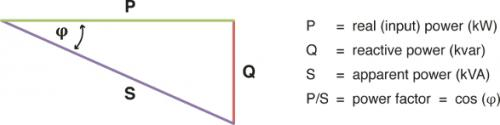Before going further, it may be helpful to review how power is used in ac circuits (see Figure 1). The real or input power (P), measured in kilowatts (kW), is the amount of power that does actual work. If the circuit has inductive loads like motors, the power required for exciting or magnetizing currents is called reactive power (Q) and is measured in kilovolt-ampere reactive (kvar) units. The combination of real power and reactive power needed by the system is the apparent power (S). The apparent power, measured in kilovolt-amperes (kVA), is proportional to the product of the voltage and total current.

Not all of the total current contributes to the real power that does the work, but utilities and industrial power operators still must size generation, transmission and distribution equipment to match the apparent power requirements of the system.

Power factor

Power factor in an ac circuit is the ratio of the real power to the apparent power.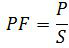As the reactive power drawn from the network decreases, the apparent power also decreases and approaches the real power. A purely resistive ac circuit would have a perfect power factor of 1.0 with zero reactive power. By contrast, a wholly inductive ac circuit theoretically could have a power factor that is very low or zero, if the real power approaches or equals zero. Although many industrial circuits have both resistive and inductive components, it will take more current to deliver the required amount of real power to a plant that has a low power factor due to induction motors.

For utilities and industrial power system operators, that means generating extra electricity to compensate for what is being wasted or lost as heat. For industrial customers, it may mean power bill surcharges and/or power quality issues on plant distribution systems. If the power factor is low enough, higher capacity wiring, switches, circuit breakers and transformers may be needed to handle the extra current.

Common ways to improve power factor

Power factor is usually improved by use of synchronous motors or power factor correction capacitors (PFCCs). In either case, reactive power is supplied locally to reduce the apparent power demand, whether the purpose is to reduce utility surcharges or, in the case of industrial power system operators, to create additional capacity. The simplest and most cost-effective way to correct existing installations is to add of PFCCs to the distribution system, giving careful consideration to capacitor ratings and locations.

Capacitor location: Figure 2 shows three possible locations for installation of PFCCs. In Location 1, the capacitors remain energized all the time. If the right inductive load is present, serious current surges (resonance) can occur, resulting in high voltage on the bus. Location 1 is recommended, however, in special cases where jogging or frequent switching occurs, such as with elevator motors, multispeed motors, open transition autotransformer starting, wye-delta (Y-Δ) starting and part winding starting (except extended or double-delta).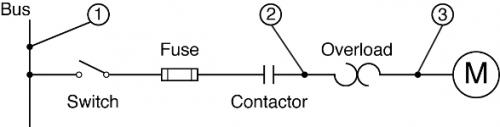Locations 2 and 3 have the advantage of the PFCCs being offline when the motor is offline. When using Location 3, however, it’s critical that the overload devices be downsized, because the capacitor reduces the circuit current through them. For this reason, Location 2 is recommended in most situations.

Capacitor sizing: When sizing the capacitors, select values that will minimize the risk of excessive voltage, as well as transient currents and torques. With the capacitors installed (see Figure 3), the reactive power (Q1) required from the bus is the difference between the motor’s reactive power demand (Q3) and the reactive power supplied by the capacitors (Q2). To balance performance against the risks associated with over-excitation, it’s typically desirable to raise the power factor to 0.90 or 0.95.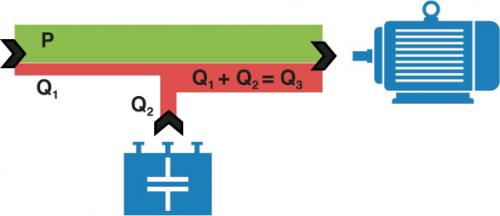As an example, consider a three-phase, 250-hp, 460-V, 277-amp, 4-pole induction motor with efficiency (eff) of 96.2% and a power factor of 0.88. To determine the capacitor rating needed to raise the power factor to 0.95, first calculate the real or input power: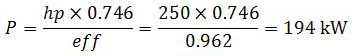Next, since the existing power factor is 0.88 = cos (φ1) and the new power factor is 0.95 = cos (φ2), we find φ1 = 28.36 deg and φ2 = 18.19 deg. The correction capacitor rating will be equal to the amount of locally generated reactive power (Q2) needed to raise the power factor to 0.95: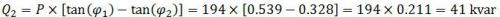For any phase angle φ1, the value tan (φ1) is the ratio of reactive to real power (kvar/kW). Thus multiplying P by the ratio Q/P above leaves Q (the reactive power). For convenience, some common values of [tan (φ1) – tan (φ2)] are supplied in Table 1.Select the nearest standard rating to the calculated value.

The effect of improved power factor

To determine the effect of improved power factor, calculate the total current (I) required from the system before and after correction: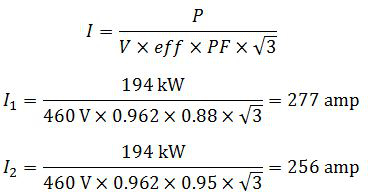For the above example, the current decreased by 21 amp, or 7.6%. It’s important to note that power factor varies with load, so these values would only be valid for the loading used for the calculations.

When considering power factor correction, it’s best to consult the motor manufacturer or a firm experienced with this modification. Doing so will ensure that the application is appropriate, the capacitors are sized correctly, and the optimum installation location is chosen.

Mike Howell is a technical support specialist at the Electrical Apparatus Service Association Inc. (EASA). EASA is an international trade association of more than 1,900 firms in 62 countries that sell and service electrical, electronic and mechanical apparatus.